0
302

# Mensuration Questions for SSC CHSL Set-3 PDF

Download SSC CHSL Mensuration questions with answers Set-3 PDF based on previous papers very useful for SSC CHSL Exams. Top-10 Very Important Maths Questions for SSC Exam

Question 1: The radius of the circumcircle of a right angled triangle is 15 cm and the radius of its inscribed circle is 6 cm. Find the sides of the triangle.

a) 30, 40, 41

b) 18, 24, 30

c) 30, 24, 25

d) 24, 36, 20

Question 2: ABCD is a rhombus. AB is produced to F and BA is produced to E such that AB = AE = BF. Then :

a) ED > CF

b) ED ⊥ CF

c) $ED^{2}+CF^{2}=EF^{2}$

d) ED ║ CF

Question 3: Two circles of same radius 5 cm, intersect each other at A and B. If AB = 8 cm, then the distance between the centres is :

a) 6 cm

b) 8 cm

c) 10 cm

d) 4 cm

Question 4: C and C are two concentric circles with centres at 0. Their radii are 12 cm. and 3 cm. respectively. B and C are the points of contact of two tangents drawn to C2 from a point A lying on the circle C1. Then the area of the quadrilateral ABOC is

a) $\frac{9\sqrt{15}}{2}$ sq.cm

b) $12\sqrt{15}$ sq.cm

c) $9\sqrt{15}$ sq.cm

d) $6\sqrt{15}$ sq.cm

Question 5: If a metallic cone of radius 30 cm and height 45 cm is melted and recast into metallic spheres of radius 5 cm, find the number of spheres.

a) 81

b) 41

c) 80

d) 40

SSC CHSL Study Material (FREE Tests)

Question 6: A cyclic quadrilateral ABCD is such that AB = BC, AD = DC, AC ⊥ BD, ∠CAD = θ. Then the angle ∠ABC =

a) $\theta$

b) $\frac{\theta}{2}$

c) $2\theta$

d) $3\theta$

Question 7: A rectangular tin sheet is 12 cm long and 5 cm broad. It is rolled along its length to form a cylinder by making the opposite edges Just to touch each other. Then the volume of the cylinder is

a) $\frac{60}{\pi}$ $cm^{3}$

b) $\frac{180}{\pi}$ $cm^{3}$

c) $\frac{120}{\pi}$ $cm^{3}$

d) $\frac{100}{\pi}$ $cm^{3}$

Question 8: On decreasing each side of an equilateral triangle by 2 cm, there is a decrease of 4√3 cm 2 in its area. The length of each side of the triangle is

a) 8 cm

b) 3 cm

c) 5 cm

d) 6 cm

Question 9: The base of a right prism is a quadrilateral ABCD. Given that AB = 9 cm, BC = 14 cm, CD = 13 cm, DA = 12 cm and ΔDAB = 90°. If the volume of the prism be 2070 cm3, then the area of the lateral surface is

a) 720 cm2

b) 810 cm2

c) 1260 cm2

d) 2070 cm2

Question 10: If the altitude of an equilateral triangle is 12√3 cm, then its area would be :

a) $12 cm^{2}$

b) $144\sqrt{3} cm^{2}$

c) $72 cm^{2}$

d) $36 \sqrt{3} cm^{2}$

Let sides be a,b,c

Since, it’s a right triangle, => Circumradius lies on the mid point of hypotenuse

=> length of hypotenuse(c) = 15*2 = 30 cm

The sum of the other two sides of a right triangle is equal to twice the sum of inradius and circumradius.

=> $(a+b) = 2(15+6)$

=> a+b = 42 ———-Eqn(1)

Now, sum of all sides = 42+30 = 72 cm

Product of the two sides(other than hypotenuse) is equal to the product of inradius and sum of all three sides.

=> ab = 6 * 72

=> ab = 432 ———Eqn(2)

From eqn(1) and (2)

=> a = 18 or b = 24 (or vice versa)

=> Sides are = 18,24,30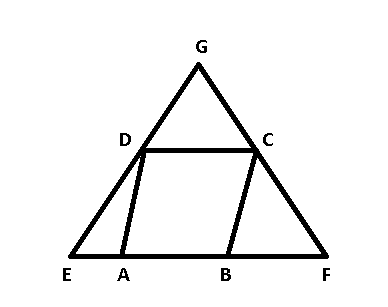ABCD is a rhombus and AB = AE = BF

=> AD = AE and BC = BF

=> $\angle$AED = $\angle$ADE and $\angle$BCF = $\angle$BFC

Let $\angle$DAB = $2\theta$ and $\angle$ABC = $2\alpha$

Using exterior angle property, we get :

=> $\angle$AED = ADE = $\theta$

and $\angle$BCF = $\angle$BFC = $\alpha$

Also, in ABCD

=> $\angle$DAB + $\angle$ABC = 180

=> $2\theta + 2\alpha = 90$

=> $\theta + \alpha = 90$

Now, in $\triangle$GEF

=> $\angle G$ + $\theta + \alpha$ = 180

=> $\angle G$ = 180 – 90 = 90

=> $ED \perp CF$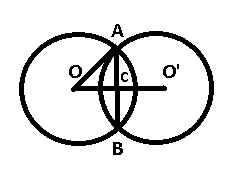AB is chord to each of the circle and AB = 8 cm

Radius of each circle = 5 cm

A line drawn from the centre of the circle perpendicular to the chord bisects it in two parts.

=> AC = 8/2 = 4 cm

Now, in $\triangle$ OAC

=> $OC = \sqrt{(OA)^2 – (AC)^2}$

=> $OC = \sqrt{25-16} = \sqrt{9}$

=> OC = 3 cm

=> OO’ = 2*3 = 6 cm

AB = AC = tangents from the same point

OB = OC = 3 and OA = 12

$\angle$ABO = 90

=> AB = $\sqrt{12^2 – 3^2} = 3\sqrt{15}$

Now, area of $\triangle$OAB = $\frac{1}{2}$ OB * AB

= $\frac{1}{2} * 3 * 3\sqrt{15} = \frac{9\sqrt{15}}{2}$

$\therefore$ area of OABC = $9\sqrt{15}$ sq. cm

Volume of metallic cone = $\frac{1}{3} \pi r^2 h$

= $\frac{1}{3} \pi * 30^2 * 45 = 13500 \pi cm^3$

Volume of sphere = $\frac{4}{3} \pi R^3$

= $\frac{4}{3} \pi * 5^3 = \frac{500 \pi}{3} cm^3$

=> Required no. of spheres = $\frac{13500 \pi}{\frac{500 \pi}{3}}$

= 81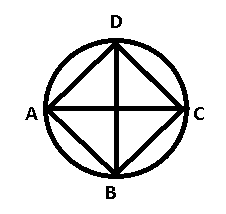AB = BC and AD = DC , $\angle CAD = \theta$

In isosceles $\triangle$ADC

=> $\angle$CAD + $\angle$ACD + $\angle$CDA = 180

=> $2\theta$ + $\angle$CDA = 180

=> $\angle$CDA = 180 – $2\theta$

Sum of opposite angles in a cyclic quadrilateral = 180

=> $\angle$CDA + $\angle$ABC = 180

=> $\angle$ABC + 180 – $2\theta$ = 180

=> $\angle$ABC = $2\theta$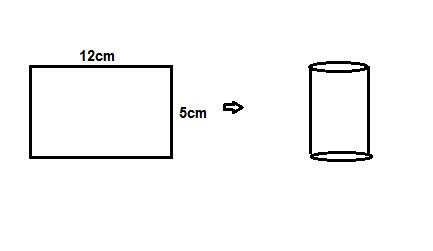From the above figure circumference of cylinder= 12cm
So 2πR= 12

R= $\frac{12}{2\pi}$

Volume of cylinder= π$(R)^{2}$h
where h and R are height and radius
So volume, V= π$(\frac{12}{2\pi})^{2}\times5$

V=$\frac{180}{\pi}$ $cm^{3}$

Area of an equilateral triangle= $(\frac{\sqrt3}{4})\times(a)^{2}$
where a is side.
When a reduced to (a-2)
Area difference = 4${\sqrt3}$
$(\frac{\sqrt3}{4})$($(a)^{2}-(a-2)^{2})$= 4${\sqrt3}$
4a-4=16
a=5 (C)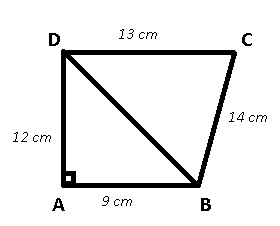In right $\triangle$DAB, using Pythagoras theorem,

=> $BD = \sqrt{(AD)^2 + (AB)^2}$

=> $BD = \sqrt{12^2 + 9^2} = \sqrt{225}$

=> $BD = 15 cm$

Now, area of $\triangle$DAB = $\frac{1}{2} * 9 * 12 = 54 cm^2$

Area of $\triangle$BCD = $\sqrt{s(s-a)(s-b)(s-c)}$

where, $s = \frac{a+b+c}{2}$

=> Area of $\triangle$BCD = $\sqrt{21 * 6 * 7 * 8} = 84 cm^2$

=> Area of quad ABCD = area of $\triangle$DAB + area of $\triangle$BCD

= 54+84 = $138 cm^2$

Volume of prism = base area * height

=> 2070 = 138 * height

=> height = 15 cm

Lateral surface area of prism = perimeter of base * height

= (12 + 9 + 14 + 13) * 15 = 48 * 15

= 720 $cm^2$

Let each side of the equilateral triangle be $a$

The altitude of an equilateral triangle bisects the opposite side.

=> $(\frac{a}{2})^2 + (12\sqrt{3})^2 = a^2$

=> $a^2 – \frac{a^2}{4} = 432$

=> $a^2 = \frac{1728}{3}$

Area of equilateral triangle = $\frac{\sqrt{3}}{4} * a^2$

= $\frac{\sqrt{3}}{4} * \frac{1728}{3}$

= $144\sqrt{3}$

We hope this Mensuration questions of set-3 pdf for SSC CHSL Exam preparation is so helpful to you.